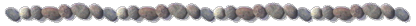Research Interests of Dr. Liao in Nonlinear Dynamics

(1)   Clean Numerical Simulation (CNS)

This is a fine numerical technique to precisely solve chaotic dynamic systems:  even the numerical noises due to truncation and round-off errors can be neglected and the chaotic solutions are reliable in a considerably long interval.

The CNS is based on the high-order Taylor expansion with high-accurate data.  For example, by means of the CNS and using 400th-order Taylor expansion and data in accuracy of 800-digit placed to the right of a decimal point, Liao obtained, for the first time, a reliable chaotic solution of Lorenz equation in a rather long interval 0 < t < 1100.  Such kind of high-precision data is possible by means of either computer algebra systems such as Mathematica and Maple, or Multiple Precision Arithmetic Library.

The CNS provides us a powerful tool to gain very accurate, reliable chaotic solutions in a considerably ling interval.  For details, please refer to the article

Liao, S.J.: On the reliability of computed chaotic solutions of non-linear differential equations.  Tellus, 61A:550 – 564 (2009)  [ arXiv:0901.2986 ]

(2)   Chaos: A bridge from micro-level uncertainty to macroscopic randomness

It is well-known that chaotic dynamic systems have the so-called sensitive dependence on initial condition (SDIC).  Due to the SDIC and the impossibility of our measuring initial conditions in arbitrary-precision, the long-term prediction of chaotic dynamic systems is impossible  - this is the famous “butterfly-effect” of chaos.

However, Lorenz equation is a simplified model from Navier-Stokes equations that is based on the so-called continuum-assumption: fluids are composed of molecules that collide with one another and solid objects. The continuum assumption, however, considers fluids to be continuous.  From the viewpoint of the continuum-assumption, the physical variables such as velocity, temperature, mass density and so on, are statistical quantities and therefore have inherent statistical fluctuation:  seriously speaking, the initial conditions of Lorenz equation contain inherent micro-level uncertainty, which has nothing to do with the measuring ability of our human being at all.

In general, such kind of micro-level uncertainty is much smaller than truncation and round-off errors of traditional numerical techniques.  Thus, traditional numerical methods are too inaccurate to investigate the propagation of such kind of micro-level uncertainty of a chaotic dynamic system with the SDIC.  Fortunately, the propagation of such kind of micro-level uncertainty can be precisely investigated by means of the CNS: using Lorenz equation as an example, it is found for the first time that, due to the SDIC of a chaotic dynamic system, the micro-level uncertainty of initial conditions transfers into the macroscopic randomness.  Thus, chaos might be a bridge from the micro-level uncertainty to macroscopic randomness.  For details, please refer to:

Liao, S.J.: Chaos – A bridge from micro-level uncertainty to macroscopic randomness.  Commun. Nonlin. Sci. Numer. Simula. Accepted (online available)  [ arXiv.1108.4472 ]

Note that chaos of Lorenz equation has close relationship to the turbulence.  So, we suggest that the turbulence is such a kind of flows of fluid, which are so instable that micro-level uncertainty transfers into macroscopic randomness.  For detailed discussion, please refer to the above-mentioned article.